# Kawamata-Viehweg vanishing theorem

Jump to: navigation, search

Letbe a connected complex projective manifold (cf. Projective scheme). Letdenote the canonical bundle of, i.e., the determinant bundle of the cotangent bundle (cf. Tangent bundle) of. A line bundleon(cf. also Vector bundle) is said to be nef if the degree of the restriction ofto any effective curve onis non-negative. A line bundle is said to be big if the sections of some positive power ofgive a birational mapping ofinto projective space. For a nef line bundleon, bigness is equivalent to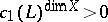, wheredenotes the first Chern class of. Letbe the dimension of theth cohomology group of the sheaf of germs of algebraic or analytic sections of an algebraic line bundleon a projective variety. The Kawamata–Viehweg vanishing theorem states that for a nef and big line bundle on a complex projective manifold,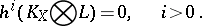Whenis a complex compact curve of genus, the bigness of a line bundleis equivalent to the line bundle being ample (cf. also Ample vector bundle), and since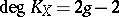, the Kawamata–Viehweg vanishing theorem takes the formif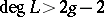; or, equivalently,if. Forwith at least one not-identically-zero section, this vanishing theorem is equivalent to the Roch identification [a15], of the number now (1998) denoted by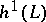with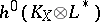, i.e., the one-dimensional Serre duality theorem. In the late 19th century, the numbersintervened in geometric arguments in much the same way as they intervene today, e.g., [a3]. For a very ample line bundleon a two-dimensional complex projective manifold, the Kawamata–Viehweg vanishing theorem was well known as the Picard theorem on the regularity of the adjoint, [a13], Vol. 2; Chap. X111; Sec. IV. This result was based on a description of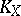[a9], Formula I.17, in terms of the double point divisor of a sufficiently general projection ofinto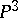.

The next large step towards the Kawamata–Viehweg vanishing theorem was due to K. Kodaira [a10]. By means of a curvature technique that S. Bochner [a2] had used to show vanishing of real cohomology groups, Kodaira showed that for an ample line bundleon a compact complex projective manifold,for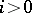. Many generalizations of the Kodaira vanishing theorem appeared. Especially notable are results of C.P. Ramanujan [a14], which include the Kawamata–Viehweg vanishing theorem in the two-dimensional case; see also [a12].

The following formulation [a6], [a7], [a4] of the Kawamata–Viehweg vanishing theorem is better adapted to applications. To state it in its simplest form, additive notation is used andis taken to be a line bundle such that, i.e., the-th tensor power of, can be written as a sum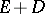of a nef and big line bundleplus an effective divisor (cf. Divisor), where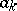are positive integers and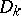are smooth irreducible divisors such that any subset of the divisors meet transversely along their intersection. Then, for,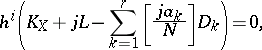where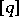denotes the greatest integer less than or equal to a real number.

For more history and amplifications of these theorems see [a5], [a16]. See [a8] and [a11] for further generalizations of the Kawamata–Viehweg vanishing theorem. The paper [a8] is particularly useful: it contains relative versions of the vanishing theorem with some singularities, for not necessarily Cartier divisors. For applications of the vanishing theorems to classical problems, see [a1].